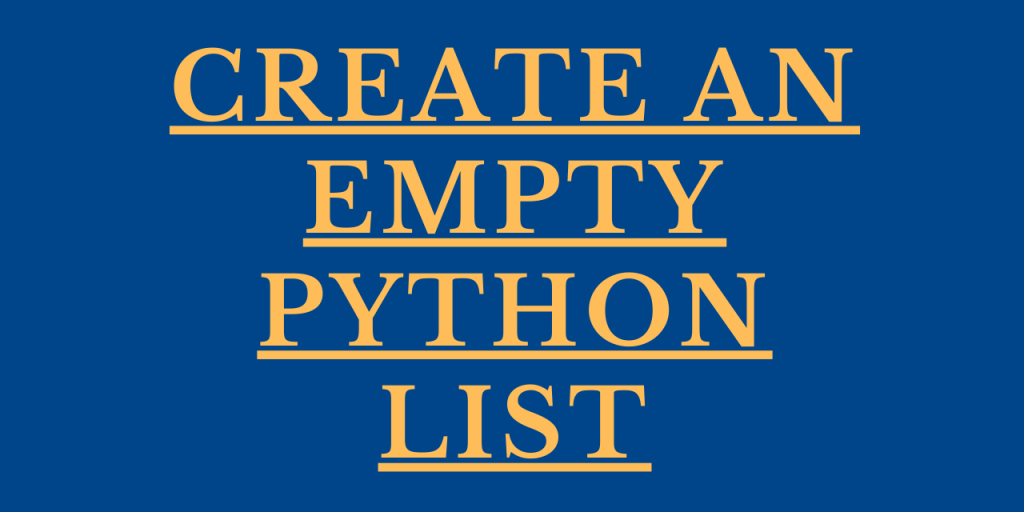# Create an Empty List in Python – 2 Easy WaysHey, all! In this article, we will be focusing on the different ways to create an empty Python List.

Let us first understand the working of Python List in detail.

## Basics of Python Lists

Python List is a dynamic data structure that stores elements into it. The beauty of a list is that different elements can be stored into it i.e. elements of different data types can be stored into it.

Example:

```lst = [1,2,4,'Python','sp']
print(lst)
```

Output:

```[1, 2, 4, 'Python', 'sp']
```

Now let us have a look at the ways to create an empty Python List.

## Technique 1: Using square brackets

We can use square brackets to create an empty list in Python. As we all know, a List uses square brackets to store its elements into it. Thus, it can be applied here as well.

Syntax:

```list = []
```

Example:

```lst = []
print("Empty list: ",lst)
```

In the above example, we have created an empty list using the square brackets.

Output:

```Empty list:  []
```

## Technique 2: Using list() function

Python list() function can be used to generate an empty list as shown below-

Syntax:

```list = list()
```

The `list() function` returns an empty list if no arguments is passed to it. However, if data values are passed to it as arguments, the list() function returns the data values in the iterable.

Example:

```lst = list()
print("Empty list: ",lst)
```

Here, we have created an empty list using the in-built list() function. As no arguments have been passed to the list() function, it returns an empty list as output.

Output:

```Empty list:  []
```

## Conclusion

By this, we have come to the end of this topic. Feel free to comment below in case you come across any questions. If you want to implement a function to check if a list is empty in Python, have a look at the article linked.

Till then, Happy Learning!!